# Vector and Scalar Values

 [ LiB ]

Physics is all about math, so it shouldn't come as a surprise that the application of physics to a game involves many mathematical structures. We'll be discussing two of these structures in this sectionscalars and vectors. If you're not a math guru, don't worrythe names may be intimidating, but they represent concepts that any programmer is familiar with, whether they know it or not. A scalar is basically a single value, like 10, 159, or 2.47. For those of you that are a bit rusty with math on a theoretical level, a scalar is analogous to a single variable in ActionScript. Defining a variable like x can only hold a single value, such as the ones listed above. The same goes for scalars.

A vector, on the other hand, represents multiple values, called components . The number of components a given vector represents is referred to as its "dimensions." For example, a 2-dimensional (or 2D ) vector represents two components; a 3D vector represents three components, and so on. Because of this, a scalar can also be referred to as a 1D vector, since both terms refer to the representation of a single value. The two components of a 2D vector are often thought of as magnitude and direction , which allow such a vector to extend from the origin of a 2D coordinate system to any distance (based on its magnitude), in any direction. See Figure 12.1.

##### Figure 12.1. The visual representation of a vector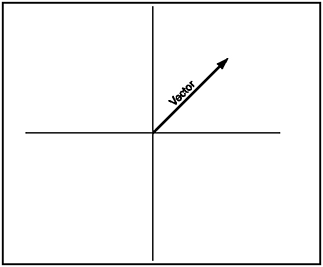Vector values are very handy when working with more complicated physics. You can add them, subtract them, and even scale them. This can help you figure out things such as the physics involved in a simple game of pool. Let's say you were programming a game of pool, and you needed to know in which direction the ball should react after it collides with another ball. Vectors can help you determine the physics involved in two billiard balls collidingwithout the use of vectors, your pool game could end up looking quite unrealistic .

#### Speed

Speed is a scalar value that represents the rate at which an object moves relative to some defined reference point. That sounds a bit geeky , so I'll restate it: Speed is the rate of change from point A to point B.

Speed is measured in meters per second (m/s). In a video game, you would have to measure speed in pixels per frame or something else that you could use to imitate the metric system. See Figure 12.2 an example.

##### Figure 12.2. Measuring speed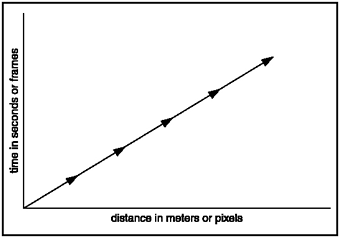#### Velocity

Velocity is a vector value that can be represented by a direction and the scalar value speed. Once you combine speed and a direction, you'll have a velocity value that is also measured in meters per second.

It is possible to plan out a direction path with vectorsthis means that you can even store how fast an object would be going on each vector segment. See Figure 12.3.

##### Figure 12.3. Tracing a vector path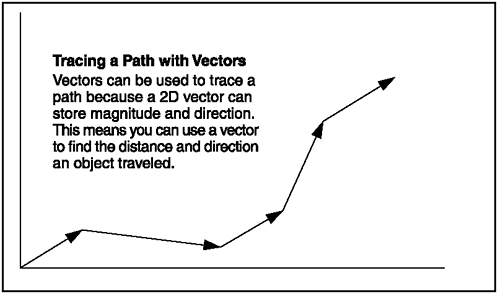I have prepared a simple demo that is very easy to understand. But this time, I want you to look at a demo with different eyes. Check out the following listing, which was extracted from GDA_PROG12.1.fla.

` // Game Development with ActionScript // By Lewis Moronta (c) 2003 // Set up the preset velocity onClipEvent(load) {   xv = 10; } // Setup ol' faithful onClipEvent(enterFrame) {   // Add the even velocity on every frame   _x += xv;   // Reverse velocities if   // we hit boundaries we don't like    if (_x > 550)     xv = -xv;   if (_x < 0)     xv = -xv; } `

There are two things that I want you to notice about this listing. One is that you can find the code within the Movie Clip, and two is that I reversed the velocities in this program just like I did in the bouncing ball demo a while back. I want you to think in terms of vectors, so check out Figure 12.4.

##### Figure 12.4. Reversing a vector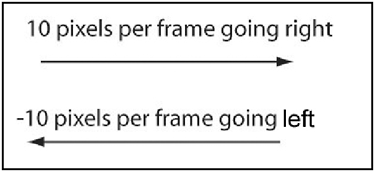As you can note from Figure 12.4, reversing a vector that is going either left or right, or east or west, is as simple as inverting its magnitude, or negating its magnitude. If your object is moving 10 pixels/frame to the right and you want it to go left, just reverse its direction by negating its velocity variableit will then be moving at -10 pixels/frame. The same rule applies for a vector moving vertically. Just remember that when dealing with horizontal or vertical, the sign of the magnitude will determine the vector's direction.

#### Acceleration

Acceleration is another vector quantity, but it's a bit deeper and harder to understand. It's the rate of change of velocity. This is why it's measured in meters per second squared (m/s/s).

Why should I even cover acceleration? Simply because nothing on this earth moves from point A to point B without speeding up or slowing down. If you can grasp and incorporate this concept in your games , you will achieve a new level of realism .

Take a look at Figure 12.5 see how the graph of a moving object is affected by accelerationone is moving with no acceleration and the other is moving with acceleration.

##### Figure 12.5. Graphed acceleration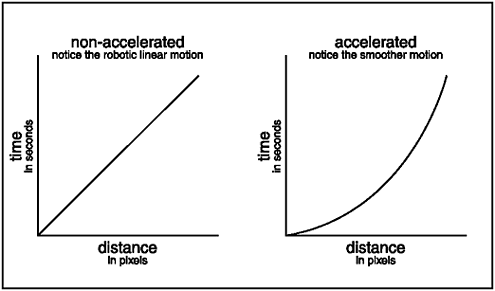GDA_PROG12.2.fla shows you the difference between a generic object with acceleration and another without. Each of the objects has its own codethe one with the acceleration was extracted to the following listing.

` // Game Development with ActionScript // By Lewis Moronta (c) 2003 onClipEvent(load) {   // Setup the acceleration   xa = 1;   // Initialize the velocity with xa   xv = xa; } // Setup ol' faithful onClipEvent(enterFrame) {   // Always add the velocity   _x += xv;   // Increase the change in velocity   xv += xa;   // Make sure we don't go overboard   if (xv > 20)     xv = 20;    // Reset   if (_x > 550) {     x = 0;     xv = 0;   } } `

The first object glides along slowly then accelerates rapidly , and then it repeats this routine. The object at the bottom of the screen moves along at a boring, steady pace. Check out the acceleration code:

` onClipEvent(load) {   // Setup the acceleration   xa = 1;   // Initialize the velocity with xa   xv = xa; } `

I set up two variables , one for the acceleration and the other for velocity. As acceleration is the change in velocity, I initialized the velocity variable with the change in velocity (acceleration).

I wrote up the code to move the object in the same way I always doby adding the velocity to the current position to move the position:

` // Always add the velocity _x += xv; `

As acceleration is the change of velocity, I changed the velocity with this line:

` // Increase the change in velocity xv += xa; `

This causesd the velocity to increase, thus making the object move fast.

To prevent any crazy speeds on the part of the object, I restricted and capped the max speed to 20 pixels per second:

` // Make sure we don't go overboard if (xv > 20)   xv = 20; `

And finally, when the object goes off the side of the screen, I wanted it to repeat the whole accelerating motion, so I reset it with the following lines:

` if (_x > 550) {   x = 0;   xv = 0; } `

You can now see how you can get creative and create a slow down. Mess around with this program and see what interesting results you can achieve.

NOTE

NOTE

I won't throw any complicated physics math at you because you most likely won't use it in your game programming ventures when you're just starting out. When you master the simple concepts I'm showing you here, I do suggest you find a good game development physics book.

 [ LiB ]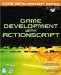Game Development with ActionScript
ISBN: 1592001106
EAN: 2147483647
Year: 2004
Pages: 162
Authors: Lewis Moronta